# 冷启动推荐模型DropoutNet深度解析与改进

+关注继续查看

## 如何解决冷启动问题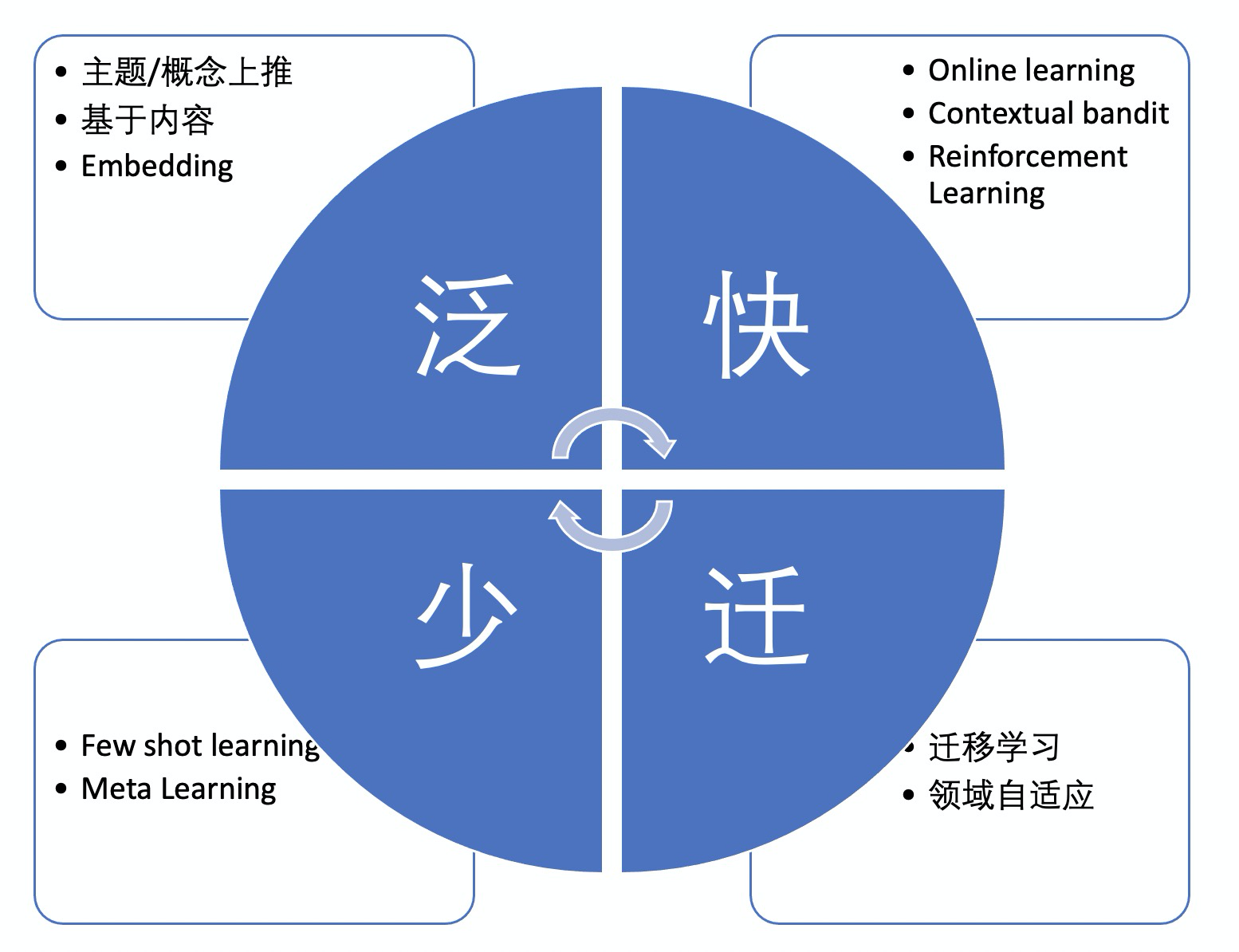：即对新物品进行泛化，在属性或主题上往更宽泛的概念上靠。比如，新上架一个商品，可以推荐给以往喜欢同品类的用户，也就是从 ”商品“ 上推至 ”品类“； 新上线一个短视频，可以推荐给关注了该视频作者的用户，也就是从 ”短视频“ 上推至 ”作者“；新发布的一篇新闻资讯，可以推荐给喜欢同一主题用户，比如把介绍”歼20“的文章推荐给一个军事迷，也就是从”新闻资讯“ 上推至 ”主题“。 本质上，这是一种基于内容的推荐（Content Based Recommandation）。当然，为了更好的推荐效果，我们有时候需要同时上推至多个不同的 ”上位概念“，比如新商品除了 上推至 ”品类“，还可以上推至 ”品牌“、”店铺“、”款式“、”颜色“等。上推的概念有时候是新物品天然就具有的，这种情况比较简单，比如商品的各种属性一般在商品发布的时候商家就填好了；也有些概念并不是本来就有，比如文章的主题，这篇文章是属于”军事“、”体育“、”美妆“ 等哪个主题是需要另外的算法来挖掘的。

：天下武功，唯快不破。所谓的冷启动物品，也就是缺少历史用户交互行为的物品，那么一个很自然的思路就是更快地收集到新物品的交互行为，并在推荐系统里加以利用。常规的推荐算法模型和数据都是以天为单位来更新，基于实时处理系统可以做到分钟级、甚至秒级的数据及模型更新。这类的方法，通常是基于强化学习/contextual bandit 类的算法。这里给两篇参考文章，就不赘述了:《Contextual Bandit算法在推荐系统中的实现及应用》、《在生产环境的推荐系统中部署Contextual bandit算法的经验和陷阱》。

：迁移学习是一种通过调用不同场景中的数据来建立模型的方法。通过迁移学习可以将知识从源域迁移到目标域。比如，新开了某个业务，只有少量样本，需要用其他场景的数据来建模。此时其他场景为源域，新业务场景为目标域。再比如，有些跨境电商平台在不同的国家有不同的站点，有些站点是新开的，只有很少的用户交互行为数据，这个时候可以用其他比较成熟的其他国家的站点的交互行为数据来训练模型，并用当前国家站点的少量样本做fine-tune，也能起到不错的冷启动效果。 使用迁移学习技术要注意的是源领域与目标领域需要具体一定的相关性，比如刚说的不同国家的站点可能卖的商品有很大一部分是重叠的。

：少样本学习（few-shot learning）技术顾名思义是只使用少量监督数据训练模型的技术。其中一直典型的少样本学习方法是元学习（meta learning）。鉴于本文的目的不是介绍这些学习技术，这样不再过多介绍，有兴趣的同学可以参考一下：《基于元学习（Meta-Learning）的冷启动推荐模型》。

1. 本文对原始DropoutNet模型进行了改造，直接使用用户与物品的交互行为数据作为训练目标进行端到端训练，从而避免了需要使用其他模型提供用户和物品的embedding作为监督信号
2. 文本创新性地提出了一种采用多种类型的损失函数的多任务学习框架，并在训练过程中使用了Negative Mining的负采样技术，在训练过程中从当前mini batch中采样负样本，扩大了样本空间，使得学习更加高效，同时适用于训练数据量比较少的场景

## DropoutNet模型解析

NIPS 2017的文章《DropoutNet: Addressing Cold Start in Recommender Systems》介绍了一种既适用于头部用户和物品，也适用于中长尾的、甚至全新的用户和物品的召回模型。

DropoutNet是一个典型的双搭结构，用户tower用来学习用户的潜空间向量表示；对应地，物品tower用来学习物品的潜空间向量表示。当用户对当前物品具有某种交互行为，比如点击、购买时，模型的损失函数设计设定用户的向量表示与物品的向量表示距离尽可能近；当给用户展现了某物品，并且用户没有对该物品产生任何交互行为时，对应的用户、物品pair构成一条负样本，模型会尽量让对应样本中用户的向量表示与物品的向量表示距离尽可能远。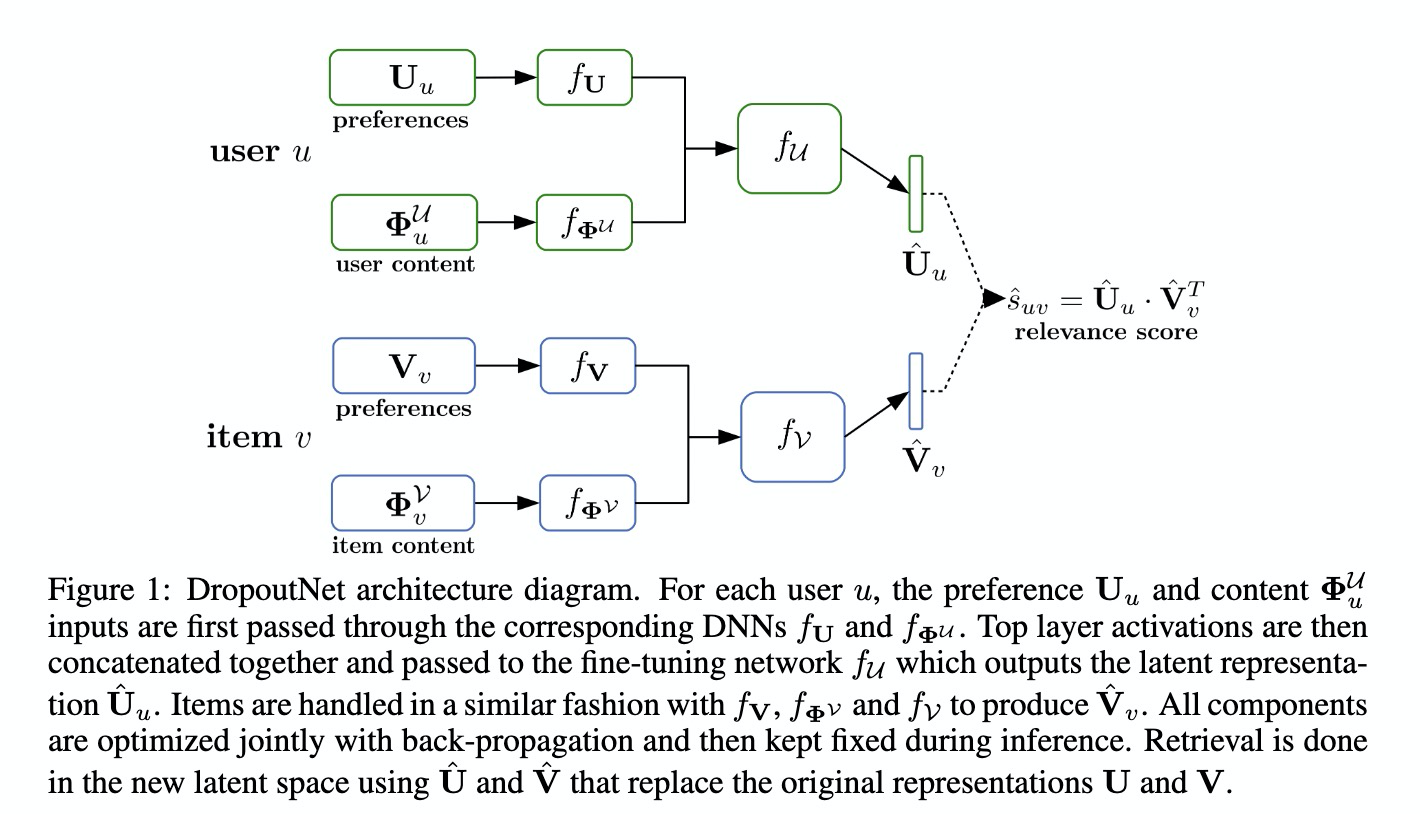O=∑u,v(UuVvT−fU(Uu,ΦuU)fV(Vv,ΦvV)T)2=∑u,v(UuVvT−U^uV^vT)2

user cold start: Ouv=(UuVvT−fU(0,ΦuU)fV(Vv,ΦvV)T)2

item cold start: Ouv=(UuVvT−fU(Uu,ΦuU)fV(0,ΦvV)T)2

DrouputNet模型的学习过程如算法1所示：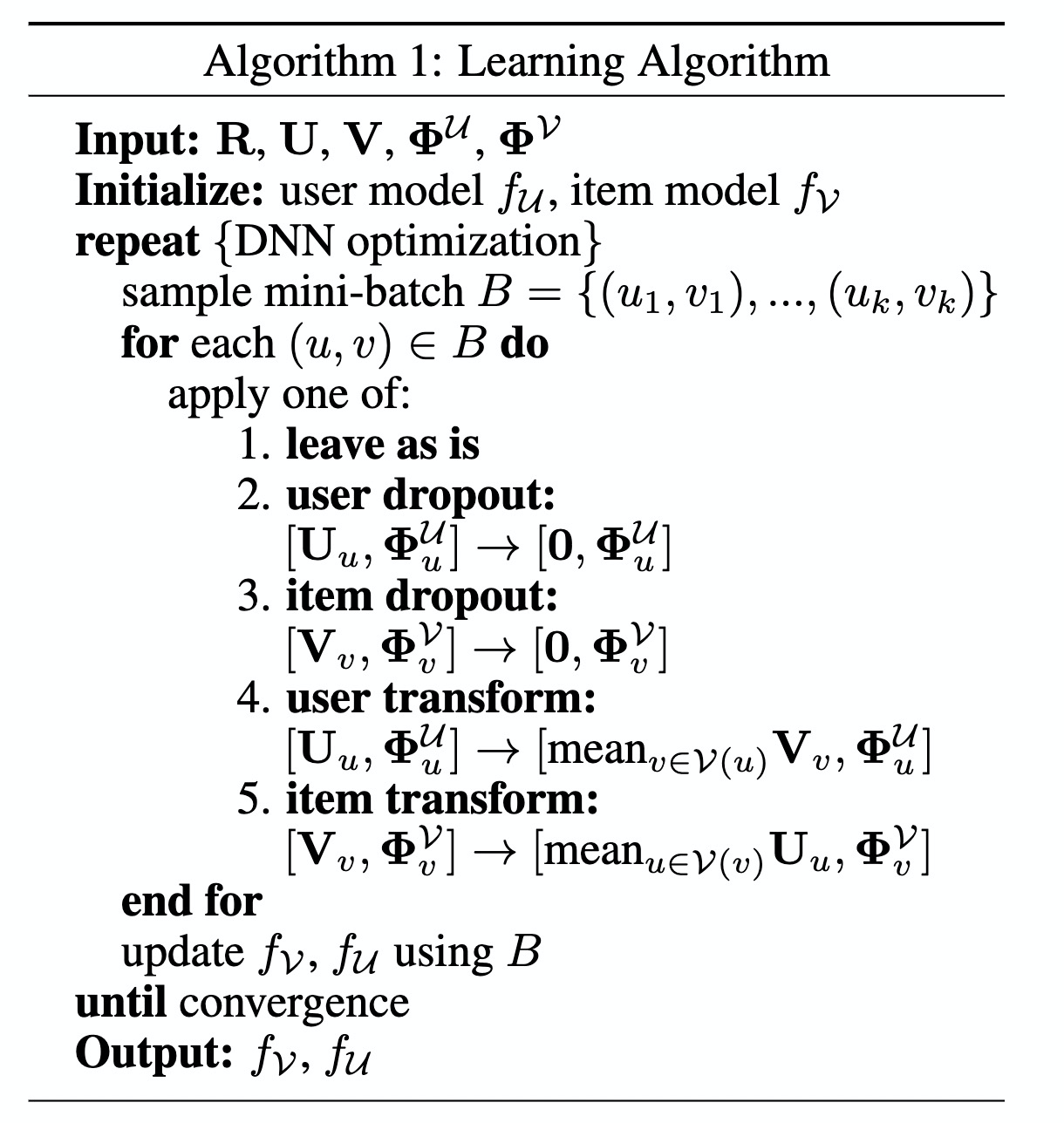## 端到端训练改造

L=−[ylog(U^uV^v+T)+(1−y)log(1−U^uV^v−T)]

## 在线负采样 & 损失函数

 负采样方法 优点 缺点 离线负采样 实现简单 样本空间有限、训练速度较慢 在线负采样 训练时动态拓展样本空间，训练较快速 实现较复杂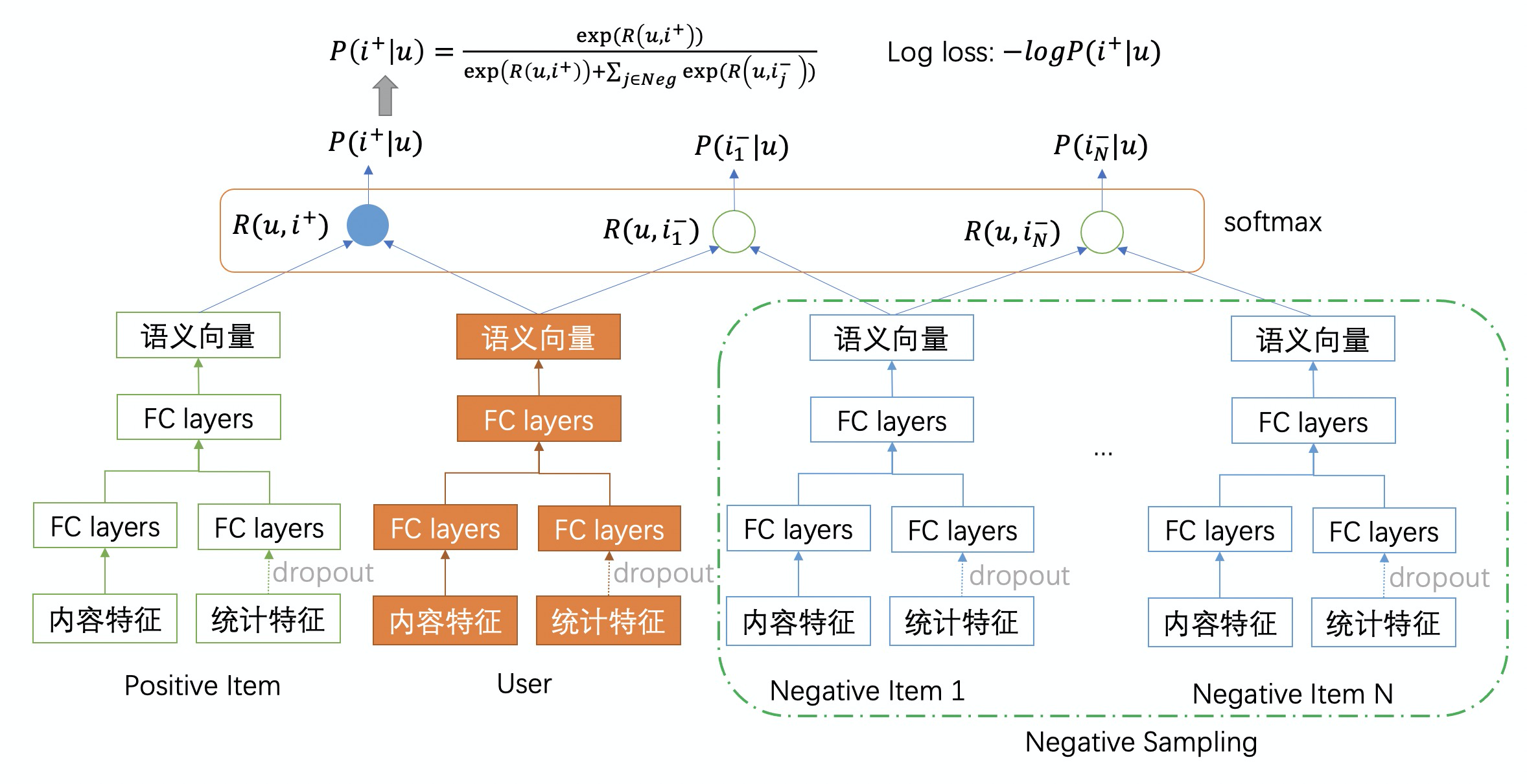L=−log(P(i+|u))=−log(exp(R(u,i+))exp(R(u,i+))+∑j∈Negexp(R(u,ij−)))

def softmax_loss_with_negative_mining(user_emb,
item_emb,
labels,
num_negative_samples=4,
embed_normed=False,
weights=1.0,
gamma=1.0,
margin=0,
t=1):
"""Compute the softmax loss based on the cosine distance explained below.
Given mini batches for user_emb and item_emb, this function computes for each element in user_emb
the cosine distance between it and the corresponding item_emb,
and additionally the cosine distance between user_emb and some other elements of item_emb
(referred to a negative samples).
The negative samples are formed on the fly by shifting the right side (item_emb).
Then the softmax loss will be computed based on these cosine distance.
Args:
user_emb: A Tensor with shape [batch_size, embedding_size]. The embedding of user.
item_emb: A Tensor with shape [batch_size, embedding_size]. The embedding of item.
labels: a Tensor with shape [batch_size]. e.g. click or not click in the session. It's values must be 0 or 1.
num_negative_samples: the num of negative samples, should be in range [1, batch_size).
embed_normed: bool, whether input embeddings l2 normalized
weights: weights acts as a coefficient for the loss. If a scalar is provided,
then the loss is simply scaled by the given value. If weights is a
tensor of shape [batch_size], then the loss weights apply to each corresponding sample.
gamma: smooth coefficient of softmax
margin: the margin between positive pair and negative pair
t: coefficient of support vector guided softmax loss
Return:
support vector guided softmax loss of positive labels
"""
batch_size = get_shape_list(item_emb)
assert 0 < num_negative_samples < batch_size, 'num_negative_samples should be in range [1, batch_size)'
if not embed_normed:
user_emb = tf.nn.l2_normalize(user_emb, axis=-1)
item_emb = tf.nn.l2_normalize(item_emb, axis=-1)
vectors = [item_emb]
for i in range(num_negative_samples):
shift = tf.random_uniform([], 1, batch_size, dtype=tf.int32)
neg_item_emb = tf.roll(item_emb, shift, axis=0)
vectors.append(neg_item_emb)
# all_embeddings's shape: (batch_size, num_negative_samples + 1, vec_dim)
all_embeddings = tf.stack(vectors, axis=1)
if isinstance(weights, tf.Tensor):
# sim_scores's shape: (num_of_pos_label_in_batch_size, num_negative_samples + 1)
sim_scores = tf.keras.backend.batch_dot(
pos_score = tf.slice(sim_scores, [0, 0], [-1, 1])
neg_scores = tf.slice(sim_scores, [0, 1], [-1, -1])
loss = support_vector_guided_softmax_loss(
pos_score, neg_scores, margin=margin, t=t, smooth=gamma, weights=weights)
return loss
def support_vector_guided_softmax_loss(pos_score,
neg_scores,
margin=0,
t=1,
smooth=1.0,
threshold=0,
weights=1.0):
"""Refer paper: Support Vector Guided Softmax Loss for Face Recognition (https://128.84.21.199/abs/1812.11317)."""
new_pos_score = pos_score - margin
cond = tf.greater_equal(new_pos_score - neg_scores, threshold)
tf.ones_like(cond, tf.float32))  # I_k
new_neg_scores = mask * (neg_scores * t + t - 1) + (1 - mask) * neg_scores
logits = tf.concat([new_pos_score, new_neg_scores], axis=1)
if 1.0 != smooth:
logits *= smooth
loss = tf.losses.sparse_softmax_cross_entropy(
tf.zeros_like(pos_score, dtype=tf.int32), logits, weights=weights)
# set rank loss to zero if a batch has no positive sample.
loss = tf.where(tf.is_nan(loss), tf.zeros_like(loss), loss)
return loss

### Pairwise Ranking

Pointwise, pairwise和listwise是LTR(Learning to Rank)领域为人熟知的三种优化目标，早在深度学习时代之前，做IR的研究者就已经发展了一系列基本方法，比较经典的工作可以参考 《Learning to Rank Using Gradient Descent》 和 《Learning to Rank- From Pairwise Approach to Listwise Approach》这两篇。

Pairwise的重要意义在于让模型的训练目标和模型实际的任务之间尽量统一。对于一个排序任务，真实的目标是让正样本的预估分数比负样本的高，对应了AUC这样的指标。在pairwise的经典论文RankNet中，pairwise的优化目标被写成了,

Cij=−yijlogPij−(1−yij)log(1−Pij)Pij=ef(xi)−f(xj)1+ef(xi)−f(xj)

def pairwise_loss(labels, logits):
pairwise_logits = tf.expand_dims(logits, -1) - tf.expand_dims(logits, 0)
logging.info('[pairwise_loss] pairwise logits: {}'.format(pairwise_logits))
tf.expand_dims(labels, -1) - tf.expand_dims(labels, 0), 0)
pairwise_pseudo_labels = tf.ones_like(pairwise_logits)
loss = tf.losses.sigmoid_cross_entropy(pairwise_pseudo_labels,
pairwise_logits)
# set rank loss to zero if a batch has no positive sample.
loss = tf.where(tf.is_nan(loss), tf.zeros_like(loss), loss)
return los

## 模型实现开源代码

EasyRec是一个易于使用的推荐算法模型训练框架，它内置了很多最先进的推荐算法模型，包括适用于推荐系统召回、排序和冷启动阶段的各种算法。可以跑在本地、DLC、MaxCompute、DataScience等多个平台上，支持从各种存储媒介（local、hdfs、maxcompute table、oss、kafka）中加载各种格式类型(text、csv、table、tfrecord)的训练和评估数据。EasyRec支持多种类型的特征，损失函数，优化器及评估指标，支持大规模并行训练。使用EasyRec，只需要配置config文件，通过命令调用的方式就可以实现训练、评估、导出、推理等功能，无需进行代码开发，帮您快速搭建推广搜算法。

EasyRec Github代码仓库：https://github.com/alibaba/EasyRec/

## 参考资料Listener、Filter、Servlet启动和销毁顺序
1050 027716 018631 0Petya勒索软件攻击预警更新：赛门铁克进一步解析Petya勒索软件

1083 0centos7网卡启动报错Job for network.service
1431 0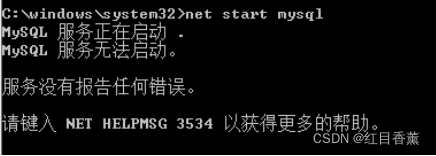net start mysql 无法启动mysql解决方案之一【NET HELPMSG 3534】
net start mysql 无法启动mysql解决方案之一【NET HELPMSG 3534】
98 019979 0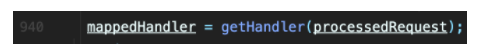SpringMVC源码解析DispatcherServlet#doDispatch方法流程（下）
SpringMVC源码解析DispatcherServlet#doDispatch方法流程（下）
30 0C# 将 Json 解析成 DateTable
#region 将 Json 解析成 DateTable /// &lt;summary&gt; /// 将 Json 解析成 DateTable。 /// Json 数据格式如: /// {table:[{column1:1,column2:2,column3:3},{column1:1,col
903 0[LeetCode] Mini Parser 迷你解析器
998 0

27

0

+ 订阅

JS零基础入门教程（上册）